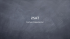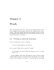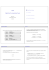# Assignment #3: Boolean Logic / Proof Techniques

## Transcription

Assignment #3: Boolean Logic / Proof Techniques
```University of Toronto at Scarborough
CSCA67—Discrete Mathematics for Computer Scientists, Fall 2014
Assignment #3: Boolean Logic / Proof Techniques
Due: November 21, 2014 at 11:59 p.m.
Warning: Your electronic submission on MarkUs affirms that this assignment is your own work and no
one else’s, and is in accordance with the University of Toronto Code of Behaviour on Academic Matters,
the Code of Student Conduct, and the guidelines for avoiding plagiarism in CSCA67.
This assignment is due by 11:59 p.m. November 21. If you haven’t finished by then, you may hand in your
assignment late with a penalty as specified in the course information sheet.

1.
In lecture we introduced the general Satisfiability Problem (SAT) and stated (without proof) that
the general SAT problem is equivalent to 3-SAT, a boolean expression in Conjunctive Normal Form
(CNF) with at most 3 terms per clause.
(a) Let A, B, C, D and E be boolean variables. Transform the following expression to CNF:
(((A ^ B) _ (C ^ D)) _ E)
(b) Is the CNF equivalent to the expression in (a) satisfiable? Prove or disprove.

2. Consider the statement if p then q which can also be written p ! q where p and q have boolean (true
or false) value. We will study the meaning of this statement. This statement is only true when either
q is true or p is false (inclusive or).
(a) Draw two circles, one which represents when p is true and one which represents when q is true.
Shade in the region that represents when p ! q is true.


(b) Repeat (a), but this time shade in the region when p ! q is false.
(c) The symbol ¬ represents not. The inverse of p ! q is ¬p ! ¬q. The converse of p ! q
is q ! p and the contrapositive of p ! q is ¬q ! ¬p. Complete the following table which
illustrates the truth value of each statement for all possible values of p and q. The first line is
done for you:
Page 1 of 2 pages.
Variables
Statement
Inverse
Converse
Contrapositive
p
q
p!q
¬p ! ¬q
q!p
¬q ! ¬p
T
T
T
T
T
T
(d) Which of inverse, converse or contrapositive is/are equivalent to the original statement? Use this
variation to prove the claim:
p
p
“If n = ab where a and b are positive integers, then a  n or b  n.”
(Hint: First show that ¬(x _ y) is equivalent to (¬x ^ ¬y).)

3. Let R, Q and Z represent the set of reals, rationals and integers, respectively. Recall that if x 2 Q
then x 2 R and x = p/q where p, q 2 Z.
Consider the claim:
“If x and y are real numbers and their product is irrational, then either x or y must be
irrational.”
(Note “irrational” means not rational.)
(a) Write this claim in logic notation. You need not use predicates, simply use xy to represent the
product of x and y, and use xy 62 Q to denote “xy is irrational”. Be careful with your quantifiers.
(b) Prove or disprove your claim in (a). First explain your proof informally (i.e., give the basic idea
behind your proof) and then formalize using an appropriate proof structure. (Hint: Work with
the contrapositive.)

4. From #3, wepsee that x 2 R is irrational if it cannot be expressed as x = p/q where p, q 2 Z.
For example, 2 is irrational. Prove that there exist irrational numbers
x and y (x and y need not be
p p2
y
distinct) such that x is rational by considering the number 2 .

5. Let X = {x1 , x2 , . . . , xn } be a set of n real numbers. Let A be the average of the numbers in X.
Prove that at least one of the xi 2 X is greater than or equal to A.
[total: 50 marks]
Page 2 of 2 pages.
```

### Algebra 1 Secondary Education MAFS.912.N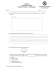### Summative Assessment-I Topper Sample Paper - 2 MATHEMATICS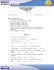### HL-511 16 oz Fluted Stadium Cup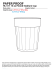### 4th Quarter - Knightsbridge Asset Management, LLC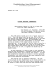### CS128 FS2011 Exam 3 Key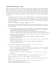### Standard: MACC.912.N-RN.2.3 Depth of Knowledge Level 2: Basic Application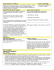### Sample Problems = +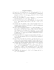### final submission form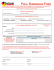### Nayelli Perez, M.S., CCDP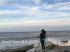### Discrete Math, Spring 2013 - Some Sample Problems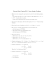### SAMPLE PAPER FOR SA-1 CLASS – X MATHEMATICS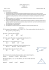### Practice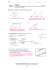### 10th Grade | Unit 2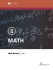### exam of last year### 2SAT - TAMU Computer Science Faculty Pages